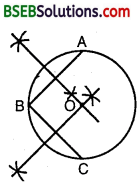# HSSlive: Plus One & Plus Two Notes & Solutions for Kerala State Board

## BSEB Class 9 Maths Chapter 10 Circles Ex 10.3 Textbook Solutions PDF: Download Bihar Board STD 9th Maths Chapter 10 Circles Ex 10.3 Book AnswersBSEB Class 9 Maths Chapter 10 Circles Ex 10.3 Textbook Solutions PDF: Download Bihar Board STD 9th Maths Chapter 10 Circles Ex 10.3 Book Answers

BSEB Class 9th Maths Chapter 10 Circles Ex 10.3 Textbooks Solutions and answers for students are now available in pdf format. Bihar Board Class 9th Maths Chapter 10 Circles Ex 10.3 Book answers and solutions are one of the most important study materials for any student. The Bihar Board Class 9th Maths Chapter 10 Circles Ex 10.3 books are published by the Bihar Board Publishers. These Bihar Board Class 9th Maths Chapter 10 Circles Ex 10.3 textbooks are prepared by a group of expert faculty members. Students can download these BSEB STD 9th Maths Chapter 10 Circles Ex 10.3 book solutions pdf online from this page.

## Bihar Board Class 9th Maths Chapter 10 Circles Ex 10.3 Books Solutions

 Board BSEB Materials Textbook Solutions/Guide Format DOC/PDF Class 9th Subject Maths Chapter 10 Circles Ex 10.3 Chapters All Provider Hsslive

## How to download Bihar Board Class 9th Maths Chapter 10 Circles Ex 10.3 Textbook Solutions Answers PDF Online?

2. Click on the Bihar Board Class 9th Maths Chapter 10 Circles Ex 10.3 Answers.
3. Look for your Bihar Board STD 9th Maths Chapter 10 Circles Ex 10.3 Textbooks PDF.
4. Now download or read the Bihar Board Class 9th Maths Chapter 10 Circles Ex 10.3 Textbook Solutions for PDF Free.

Find below the list of all BSEB Class 9th Maths Chapter 10 Circles Ex 10.3 Textbook Solutions for PDF’s for you to download and prepare for the upcoming exams:

## BSEB Bihar Board Class 9th Maths Solutions Chapter 10 Circles Ex 10.3

Question 1.
Draw different pairs of circles. How many points does each pair have in common? What is the maximum number of common points?
Solution:
On drawing a different pairs of circles, we find that they have two points in common. Now, we shall prove that a pair of circle cannot intersect each other at more than two points. Let the two circles cut each other at three points. But from the three points only one circle passes.

Hence, two circles if intersect each other then they will intersect at two points. These two points are the ends of their common chords. This chord can be a common chord of a number of circles which will pass through the end points of this common chord.

Question 2.
Suppose you are given a construction to find its centre.
Solution:
Steps of Construction :
1. Take 3 points A, B and C on the circumference of the circle.
2. Join AB and BC.3. Draw PQ and RS, the perpendicular bisectors of AB and BC, which intersect each other at O. Then, O is the centre of the circle.

Question 3.
If two circles intersect at two points, prove that their centres lie on the perpendicular bisector of the common chord.
Solution:
Given : Two circles, with centres O and O’ intersect at two points A and B so that AB is the common chord of the two circles and 00′ is the line segment joining the centres of the two circles. Let OO’ intersect AB at M.
To prove : OO’ is the perpendicular bisector of AB.
Construction : Draw line segments OA, OB, O’A and O’B.Proof:
In As OAO’ and OBO
OA = OB [Radii of the same circle]
O’A = O’B [Radii of the same circle]
and; OO’ = OO’
By SSS criterion of congruence, we have
∆ OAO’ ≅ ∆ OBO’
⇒ ∠AO O’ = ∠BOO’
⇒ ∠AOM = ∠BOM …(1) [∵ ∠AOO’ = ∠AOM and ∠BOM = ∠BOO’]
In ∆s AOM and BOM, we have
OA = OB [Radii of the same circle]
∠AOM = ∠BOM [From (1)]
and, OM = OM [Common]
∴ By SAS criterion of congruence, we have
∆ AOM ≅ ∆ BOM
⇒ AM = BM and ∠AMO = ∠BMO
But ∠AMO + ∠BMO = 180°
∴ ∠AM0 = 180° ⇒ ∠AMO = 90°
Thus, AM = BM and ∠AMO = ∠BMO = 90°
Hence, OO’ is the perpendicular bisector of AB.

## Bihar Board Class 9th Maths Chapter 10 Circles Ex 10.3 Textbooks for Exam Preparations

Bihar Board Class 9th Maths Chapter 10 Circles Ex 10.3 Textbook Solutions can be of great help in your Bihar Board Class 9th Maths Chapter 10 Circles Ex 10.3 exam preparation. The BSEB STD 9th Maths Chapter 10 Circles Ex 10.3 Textbooks study material, used with the English medium textbooks, can help you complete the entire Class 9th Maths Chapter 10 Circles Ex 10.3 Books State Board syllabus with maximum efficiency.

## FAQs Regarding Bihar Board Class 9th Maths Chapter 10 Circles Ex 10.3 Textbook Solutions

#### Can we get a Bihar Board Book PDF for all Classes?

Yes you can get Bihar Board Text Book PDF for all classes using the links provided in the above article.

## Important Terms

Bihar Board Class 9th Maths Chapter 10 Circles Ex 10.3, BSEB Class 9th Maths Chapter 10 Circles Ex 10.3 Textbooks, Bihar Board Class 9th Maths Chapter 10 Circles Ex 10.3, Bihar Board Class 9th Maths Chapter 10 Circles Ex 10.3 Textbook solutions, BSEB Class 9th Maths Chapter 10 Circles Ex 10.3 Textbooks Solutions, Bihar Board STD 9th Maths Chapter 10 Circles Ex 10.3, BSEB STD 9th Maths Chapter 10 Circles Ex 10.3 Textbooks, Bihar Board STD 9th Maths Chapter 10 Circles Ex 10.3, Bihar Board STD 9th Maths Chapter 10 Circles Ex 10.3 Textbook solutions, BSEB STD 9th Maths Chapter 10 Circles Ex 10.3 Textbooks Solutions,
Share: